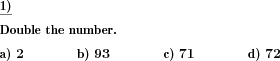Custom math worksheets at your fingertipsDetails for problem "Double a given number"

Quickname: 4627

Elementary School, Primary School, Junior High School, Middle School, High School.

Summary

Double a number with or without decimal places.

ExampleDescription

This exercise is about doubling numbers. In particular, the concept of doubling as multiplication by two is the main focus here. Therefore, the problem definition is deliberately kept short. The request "double" stands for itself and the meaning for the arithmetic task, which follows from it, must be developed. Both whole numbers and decimal fractions, i.e. numbers with digits after the decimal point, can be given for doubling.

The numbers can lie in a selectable number range, in which the outcome will also fall. The number of decimal places is adjustable.

The number of numbers to be doubled in this task can also be set.

Download free printable worksheets for this math problem here. The worksheet contains the problems only, the solution sheet includes the answers. Just click on the respective link.

•Worksheet 1Solution sheet with answers
•Worksheet 2Solution sheet with answers
•Worksheet 3Solution sheet with answers

If you can not see the solution sheets for download, they may be filtered out by an ad blocker that you may have installed. If this is the case, please allow ads for this page and reload the page. The solution sheets will then reappear.

• Do these sample worksheets do not really fit?
• Do you need more math worksheets, with a different level of difficulty?
• Would you like to combine different problems on a worksheet and adjust them to your needs?
• As a teacher, you can put together your own worksheets using the automatically generated math problems provided.
With a free initial credit, you can start creating your own math worksheets in a few minutes.

You can try it for free! Register here, to create custom worksheets now!

Customization options for this problem

Parameter
Possible values
Number of problems
1, 2, 3, 4, 5, 6, 7, 8, 9, 10
Number range
10, 20, 30, 40, 50, 100, 200, 500, 1000, 10000, 100000
Decimal places
0, 1, 2, 3Deutsche Version dieser Aufgabe
These informational pages with samples describe math problems that can be combined on custom math worksheets with solutions for home and K-12 school use.
Deutsche Seiten
×